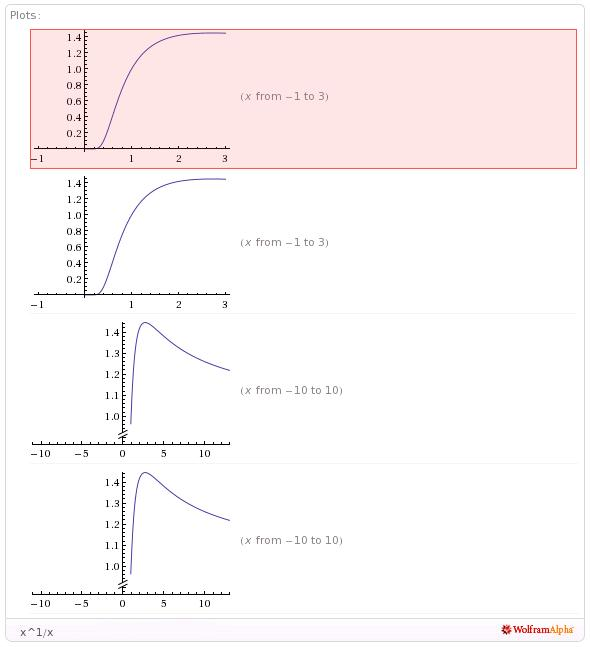# inmo question:

Determine the largest number in the infinite sequence

1^1/1 , 2^1/2 , 3^1/3 , 4^1/4 , ............ n^1/n

•man111 singh ·

I am getting \hspace{-16}\mathbf{3^{\frac{1}{3}}}\$

•Arnab Kundu ·

Yes it is.
Proof: Let a function f(x)=x^{\frac{1}{x}}
The maxima will occur at the point where f'(x)=0 and f"(x)<0
We get,
\frac{d}{dx}(f(x))=x^{\frac{1}{x}-2}(1-\ln x)
So, f'(x)=0 for x=eâ‰ˆ2.71...
And we can prove f"(x)<0

Since 3 is the closest integer to e 3^(1/3) sholud be least.
and you can also check the graph.•ameyaloya ·

plz solve this question without using concepts of differentiation....

i dont know calculas...

•Lokesh Verma ·

n(n+1) â‰ˆ (n+1)n

n â‰ˆ (1+1/n)n

n â‰ˆ 1 + 1 + terms less than 1 each...

if u see the last 2 terms they will together be less than 1 when n is greater than 3

so it is clearn that LHS is greater than RHS

so we can say

n(n+1) > (n+1)n
if n >3

by simple check we can get that among n = 1, 2, 3 n=3 is the largest

•Shubhodip ·

ameyaloya have u cleared RMO 2?

are you from pune?

in which yr did it appear in INMO?

•ameyaloya ·

@ shubhodip

yes i cleared rmo2.

i m not from pune ; i live in aurangabad in maharashtra.

this question nappeared in inmo 1990.

•Shubhodip ·

ok, but you wrote rmo from pune region i guess ...

•ameyaloya ·

@ shubhodip

yes.....but why???

•